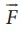Home | | Physics 11th std | Gravitational potential V(r)

# Gravitational potential V(r)

The gravitational potential at a distance r due to a mass is defined as the amount of work required to bring unit mass from infinity to the distance r and it is denoted as V(r).

Gravitational potential V(r)

It is explained in the previous sections that the gravitational fielddepends only on the source mass which creates the field. It is a vector quantity. We can also define a scalar quantity called â€śgravitational potentialâ€ť which depends only on the source mass.

The gravitational potential at a distance r due to a mass is defined as the amount of work required to bring unit mass from infinity to the distance r and it is denoted as V(r). In other words, the gravitational potential at distance r is equivalent to gravitational potential energy per unit mass at the same distance r. It is a scalar quantity and its unit is Jâ€†kg-1We can determine gravitational potential from gravitational potential energy. Consider two masses m1 and m2 separated by a distance r which has gravitational potential energy U(  r ) (Figure 6.15). The gravitational potential due to mass m1 at a point P which is at a distance r from m1 is obtained by making m2 equal to unity (m2 = 1kg). Thus the gravitational potential V ( r ) due to mass m1 at a distance r isGravitational field and gravitational force are vector quantities whereas the gravitational potential and gravitational potential energy are scalar quantities. The motion of particles can be easily analyzed using scalar quantities than vector quantities. Consider the example of a falling apple:

Figure 6.16 shows an apple which falls on Earth due to Earthâ€™s gravitational force. This can be explained using the concept of gravitational potential V( r ) as follows.The gravitational potential V( r ) at a point of height h from the surface of the Earth is given by,The gravitational potential Vr on the surface of Earth is given by,Thus we see thatIt is already discussed in the previous section that the gravitational potential energy near the surface of the Earth at height h is mgh. The gravitational potential at this point is simply V ( h ) = U ( h) /m = gh. In fact, the gravitational potential on the surface of the Earth is zero since h is zero. So the apple falls from a region of a higher gravitational potential to a region of lower gravitational potential. In general, the mass will move from a region of higher gravitational potential to a region of lower gravitational potential.

### EXAMPLE 6.5

Water falls from the top of a hill to the ground. Why?

This is because the top of the hill is a point of higher gravitational potential than the surface of the Earth i.e.Vhill > VgroundThe motion of particles can be analyzed more easily using scalars like U ( r )or V(r)  than vector quantities likeor. In modern theories of physics, the concept of potential plays a vital role.

### EXAMPLE 6.6

Consider four masses m1, m2, m3, and m4 arranged on the circumference of a circle as shown in figure belowCalculate

a) The gravitational potential energy of the system of 4 masses shown in figure.

b) The gravitational potential at the point O due to all the 4 masses.

Solution

The gravitational potential energy U( r ) can be calculated by finding the sum of gravitational potential energy of each pair of particles.If all the masses are equal, then m1 = m2 = m3 = m4 = MThe gravitational potential V(r) at a point O is equal to the sum of the gravitational potentials due to individual mass. Since potential is a scalar, the net potential at point O is the algebraic sum of potentials due to each mass.Study Material, Lecturing Notes, Assignment, Reference, Wiki description explanation, brief detail
11th Physics : UNIT 6 : Gravitation : Gravitational potential V(r) |